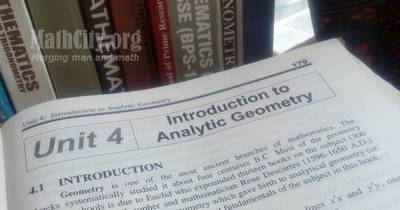# Unit 04: Introduction to Analytic GeometryNotes (Solutions) of Unit 04: Introduction to Analytic Geometry, Calculus and Analytic Geometry, MATHEMATICS 12 (Mathematics FSc Part 2 or HSSC-II), Punjab Text Book Board Lahore. You can view online or download PDF. To view PDF, you must have PDF Reader installed on your system and it can be downloaded from Software section.

• Introduction
• The Distance Formula
• Point Dividing the Join of Two Points in a given Ratio
• Exercise 4.1
• Translation and Rotation of Axes
• Exercise 4.2
• Equations of Straight Lines
• Slope or Gradient of a Straight Line Joining Two Points
• Equation of a Straight Lines Parallel to the x-axis (or Perpendicular to the y-axis)
• Equation of a Straight Lines Parallel to the y-axis (or Perpendicular to the x-axis)
• Derivation of Standard forms of Equations of Straight Lines
• A Linear Equation in two Variables Represents a Straight Line
• To Transform the General Linear Equation in Standard Forms
• Position of a point with respect to a line
• Two and Three Straight Lines
• The Points of Intetrsection of two Straight Lines
• Condition of Concurrency of Three Straight Lines
• The Equation of Lines through the Point of Intersection of two Lines
• Distance of a Point from a Line
• Distance between two Parallel Lines
• Area of a Triangular Region whose Vertices are given
• Exercise 4.3
• Angle Between Two Lines
• Equation of a Straight Line in Matrix form
• Exercise 4.4
• Homogeneous Equation of the Second Degree in two Veriables
• Homogeneous Equation
• To find measure of the angle between the lines represented by $ax^2+ 2hxy+by^2=0$
• Exercise 4.5
• Exercise 4.5 | View Online | Download PDF (168KB)• fsc/fsc_part_2_solutions/ch04